Get inspired by the success stories of our students in IIT JAM MS, ISI  MStat, CMI MSc DS.  Learn More

# ISI MStat PSB 2018 Problem 1 | System of Linear EquationsThis is a beautiful sample problem from ISI MStat PSB 2018 Problem 1. This is based on finding the real solution of a system of homogeneous equations. We provide a detailed solution with prerequisites mentioned explicitly.

## Problem- ISI MStat PSB 2018 Problem 1

Find all real solutions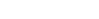for the system of equations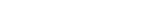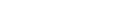## Solution

We are given the system of homogeneous equations as follows ,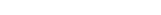Let ,As A is a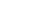matrix so ,if the Rank of A is <3 then it has infinitely many solution and

if Rank of A is 3 then it has only trivial solution i.e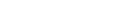Let's try to find the rank of matrix A from it's row echelon form ,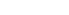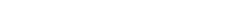Now see when the determinant of A =0 as then the Rank(A) will be <3 and then it has infinitely many solutions

Det(A)=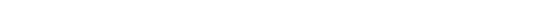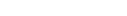So, if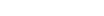then Rank(A) <3 hence it has infinitely many solutions

Now from here we can say that if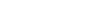then Rank (A) =3 then the system of homogeneous equations has only trivial solution i.eFor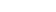system of homogeneous equation is as follows ,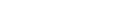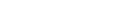Solving this we get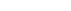and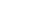. Hence solution space is {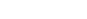} ,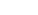.

Similarly , for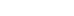we have solution space {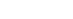} and {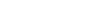} respectively .

Therefore , real solutions (x1,x2,x3,λ) for the system of equations are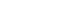,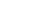and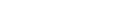,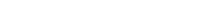.

## Previous ISI MStat Posts:

This is a beautiful sample problem from ISI MStat PSB 2018 Problem 1. This is based on finding the real solution of a system of homogeneous equations. We provide a detailed solution with prerequisites mentioned explicitly.

## Problem- ISI MStat PSB 2018 Problem 1

Find all real solutionsfor the system of equations## Solution

We are given the system of homogeneous equations as follows ,Let ,As A is amatrix so ,if the Rank of A is <3 then it has infinitely many solution and

if Rank of A is 3 then it has only trivial solution i.eLet's try to find the rank of matrix A from it's row echelon form ,Now see when the determinant of A =0 as then the Rank(A) will be <3 and then it has infinitely many solutions

Det(A)=So, ifthen Rank(A) <3 hence it has infinitely many solutions

Now from here we can say that ifthen Rank (A) =3 then the system of homogeneous equations has only trivial solution i.eForsystem of homogeneous equation is as follows ,Solving this we getand. Hence solution space is {} ,.

Similarly , forwe have solution space {} and {} respectively .

Therefore , real solutions (x1,x2,x3,λ) for the system of equations are,and,.

## Previous ISI MStat Posts:

This site uses Akismet to reduce spam. Learn how your comment data is processed.

### Knowledge Partner